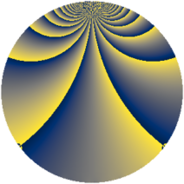# Properties

 Label 1148.2.wLevel $1148$ Weight $2$ Character orbit 1148.w Rep. character $\chi_{1148}(489,\cdot)$ Character field $\Q(\zeta_{8})$ Dimension $112$ Sturm bound $336$

# Learn more about

## Defining parameters

 Level: $$N$$ $$=$$ $$1148 = 2^{2} \cdot 7 \cdot 41$$ Weight: $$k$$ $$=$$ $$2$$ Character orbit: $$[\chi]$$ $$=$$ 1148.w (of order $$8$$ and degree $$4$$) Character conductor: $$\operatorname{cond}(\chi)$$ $$=$$ $$287$$ Character field: $$\Q(\zeta_{8})$$ Sturm bound: $$336$$

## Dimensions

The following table gives the dimensions of various subspaces of $$M_{2}(1148, [\chi])$$.

Total New Old
Modular forms 696 112 584
Cusp forms 648 112 536
Eisenstein series 48 0 48

## Trace form

 $$112q - 16q^{9} + O(q^{10})$$ $$112q - 16q^{9} - 24q^{15} - 4q^{21} - 8q^{29} + 48q^{37} + 8q^{43} - 24q^{49} + 48q^{51} + 112q^{57} + 8q^{63} + 56q^{65} - 16q^{67} + 32q^{71} + 4q^{77} + 8q^{79} - 16q^{85} - 84q^{91} - 32q^{93} + 48q^{95} - 16q^{99} + O(q^{100})$$

## Decomposition of $$S_{2}^{\mathrm{new}}(1148, [\chi])$$ into newform subspaces

The newforms in this space have not yet been added to the LMFDB.

## Decomposition of $$S_{2}^{\mathrm{old}}(1148, [\chi])$$ into lower level spaces

$$S_{2}^{\mathrm{old}}(1148, [\chi]) \cong$$ $$S_{2}^{\mathrm{new}}(287, [\chi])$$$$^{\oplus 3}$$$$\oplus$$$$S_{2}^{\mathrm{new}}(574, [\chi])$$$$^{\oplus 2}$$